Next: 4.2.2 Discretization of the Up: 4.2 Discretization of the Previous: 4.2 Discretization of the

## 4.2.1 Discretization of Laplace's Equation

The electric potential is calculated from equation (3.71). Multiplying it by a test function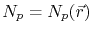, and integrating over the domain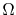one obtains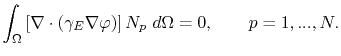(4.25)

Using Green's formula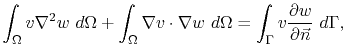(4.26)

where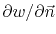represents the normal derivative in the outward normal direction to the boundary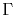, and assuming a Neumann boundary condition, i.e. vanishing normal derivatives, on, equation (4.25) can be written as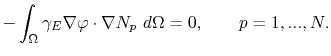(4.27)

Since the electrical conductivity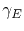depends on the temperature according to (3.73) and varies along the simulation domain, it is part of the integrand in (4.27). However, in a single element the conductivity is assumed to be constant, being determined by the average of the temperature on the element nodes, i.e.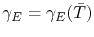with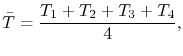(4.28)

for a tetrahedral element. Applying the discretization for the electric potential as in (4.6),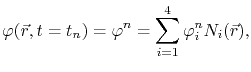(4.29)

where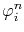is the electric potential of the node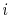at a time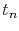, equation (4.27) for a single element becomes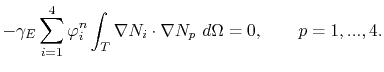(4.30)

Using the shorthand notation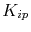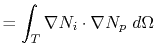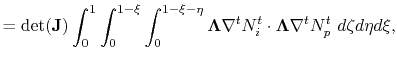(4.31)

where the last term is the calculation in the transformed coordinate system, (4.30) is rewritten as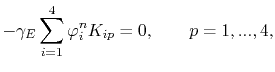(4.32)

which corresponds to a discrete system of 4 equations with 4 unknowns, i.e. the electric potential at each node of the tetrahedral element.Next: 4.2.2 Discretization of the Up: 4.2 Discretization of the Previous: 4.2 Discretization of the

R. L. de Orio: Electromigration Modeling and Simulation